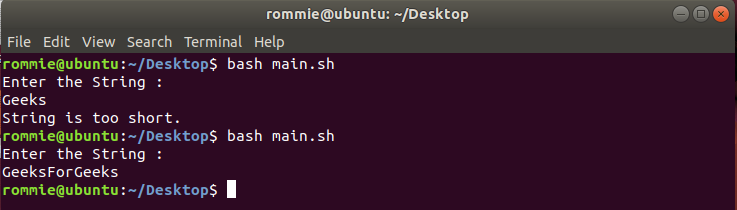Related Articles

# Shell Script To Check the String For a Particular Condition

• Last Updated : 14 Sep, 2021

Here, we are going to write a shell script that will echo (print) a suitable message if the accepted string does not meet a particular condition. Suppose we have given some certain conditions then our task is to print the message if the accepted string doesn’t match the condition.

Example 1:

If the accepted string’s length is smaller than 10, we will display a message saying “String is too short“.

```Input:  "abcdef"
Output:  String is too short
Length is 6, which is smaller than 10.```

Approach:

• We will take a string from the user
• We have to find the length of the string so that we can decide whether it is smaller or larger than 10.
• We will find the length using the following command:
`len=`echo -n \$str | wc -c``

Below is the Implementation:

```# Shell script to print message
# when condition doesn't matched

# taking user input
echo "Enter the String : "

# Finding the length of string
# -n flag to avoid new line
# wc -c count the chars in a file
len=`echo -n \$string | wc -c`

# Checking if length is smaller
# than 10 or not
if [ \$len -lt 10 ]
then
# if length is smaller
# print this message
echo "String is too short."
fi```

Output:Example 2: We will accept two input String and find whether both are equal or not.

String1 -> a = “abc”

String2 -> b = “abc”

Here a and b are equal.

Below is the implementation:

```# Shell script to check whether two
# input strings is equal

# take user input
echo "Enter string a : "

echo "Enter string b : "

# check equality of input
if [ "\$a" = "\$b" ]; then

# if equal print equal
echo "Strings are equal."
else
# if not equal
echo "Strings are not equal."
fi```

Output:

```Geeks
Geeks
Strings are equal.```

Example 3:

We are working with strings, so now we will take two strings as input and check which of the two is greater in respect of lexicographical (alphabetical) order.

string1 > string2 : The greater than operator returns true if the left operand is greater than the right sorted by lexicographical (alphabetical) order.

string1 < string2 : The less than operator returns true if the right operand is greater than the right sorted by lexicographical (alphabetical) order.

```# Shell script to check which
# string is lexicographically
# larger

# user input
echo "Enter String a : "

echo "Enter String b : "

# if a is greater than b
if [[ "\$a" > "\$b" ]]; then
echo "\${a} is lexicographically greater then \${b}."

# if b is greater than a
elif [[ "\$a" < "\$b" ]]; then
echo "\${b} is lexicographically greater than \${a}."

# if both are equal
else
echo "Strings are equal"
fi```

Output:

```abc
def
def is lexicographically greater than abc.```
My Personal Notes arrow_drop_up